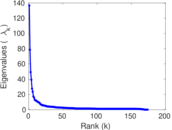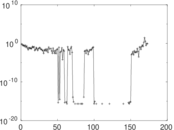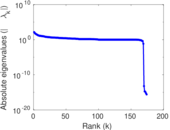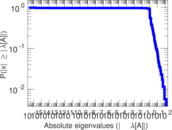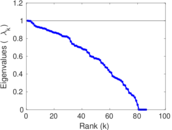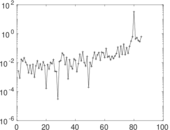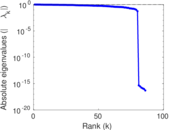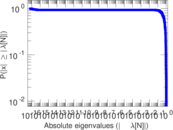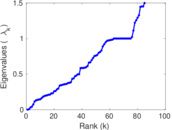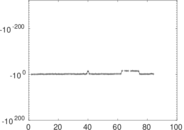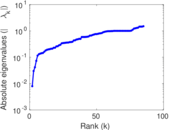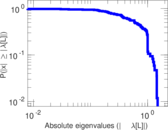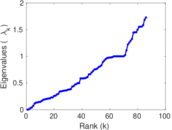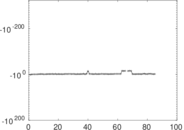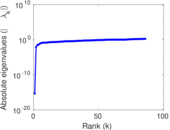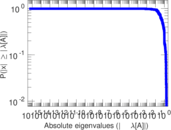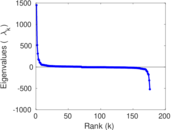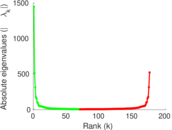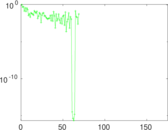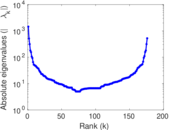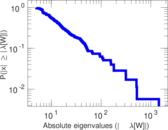# Wiktionary edits (ln)

This is the bipartite edit network of the Lingala Wiktionary. It contains users and pages from the Lingala Wiktionary, connected by edit events. Each edge represents an edit. The dataset includes the timestamp of each edit.

 Code `mln` Internal name `edit-lnwiktionary` Name Wiktionary edits (ln) Data source http://dumps.wikimedia.org/ AvailabilityDataset is available for download Consistency checkDataset passed all tests Category Authorship network Dataset timestamp 2017-10-20 Node meaning User, article Edge meaning Edit Network formatBipartite, undirected Edge typeUnweighted, multiple edges Temporal dataEdges are annotated with timestamps

## Statistics

 Size n = 1,449 Left size n1 = 176 Right size n2 = 1,273 Volume m = 4,895 Unique edge count m̿ = 2,880 Wedge count s = 448,600 Claw count z = 69,974,774 Cross count x = 8,902,741,461 Square count q = 302,872 4-Tour count T4 = 4,223,444 Maximum degree dmax = 1,507 Maximum left degree d1max = 1,507 Maximum right degree d2max = 114 Average degree d = 6.756 38 Average left degree d1 = 27.812 5 Average right degree d2 = 3.845 25 Fill p = 0.012 854 4 Average edge multiplicity m̃ = 1.699 65 Size of LCC N = 1,097 Diameter δ = 14 50-Percentile effective diameter δ0.5 = 3.374 29 90-Percentile effective diameter δ0.9 = 7.222 56 Median distance δM = 4 Mean distance δm = 4.241 24 Gini coefficient G = 0.733 832 Balanced inequality ratio P = 0.214 096 Left balanced inequality ratio P1 = 0.098 672 1 Right balanced inequality ratio P2 = 0.289 070 Relative edge distribution entropy Her = 0.774 279 Power law exponent γ = 2.566 70 Tail power law exponent γt = 3.051 00 Tail power law exponent with p γ3 = 3.051 00 p-value p = 0.000 00 Left tail power law exponent with p γ3,1 = 1.721 00 Left p-value p1 = 0.276 000 Right tail power law exponent with p γ3,2 = 3.681 00 Right p-value p2 = 0.002 000 00 Degree assortativity ρ = −0.067 128 7 Degree assortativity p-value pρ = 0.000 312 146 Spectral norm α = 136.716 Algebraic connectivity a = 0.007 927 18 Spectral separation |λ1[A] / λ2[A]| = 1.741 08 Controllability C = 1,087 Relative controllability Cr = 0.762 807

## Plots

### Fruchterman–Reingold graph drawing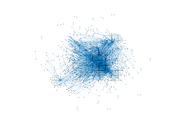### Degree distribution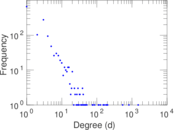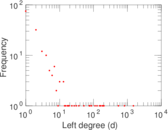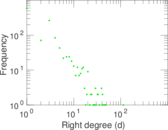### Cumulative degree distribution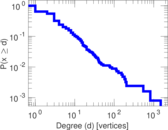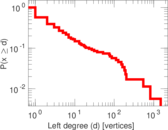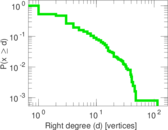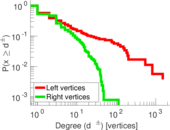### Lorenz curve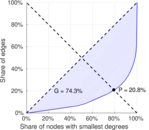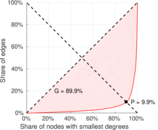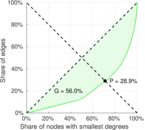### Spectral distribution of the adjacency matrix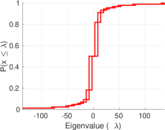### Spectral distribution of the normalized adjacency matrix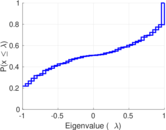### Spectral distribution of the Laplacian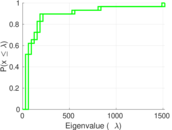### Spectral graph drawing based on the adjacency matrix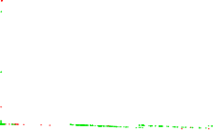### Spectral graph drawing based on the Laplacian### Spectral graph drawing based on the normalized adjacency matrix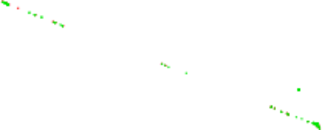### Degree assortativity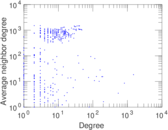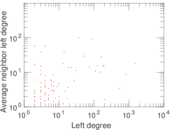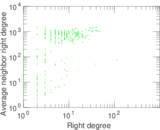### Zipf plot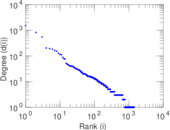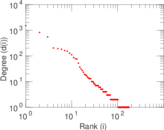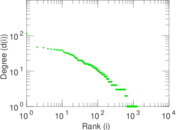### Hop distribution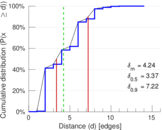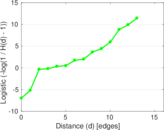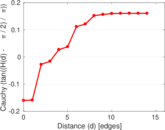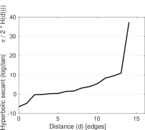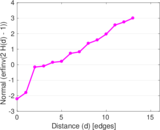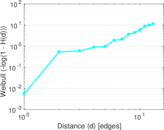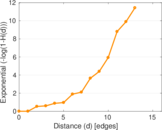### Double Laplacian graph drawing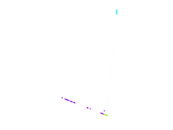### Delaunay graph drawing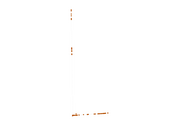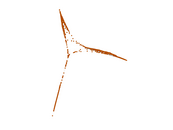### Edge weight/multiplicity distribution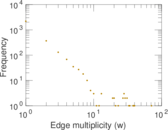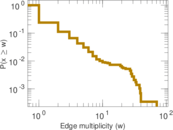### Temporal distribution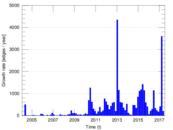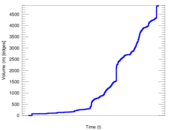### Temporal hop distribution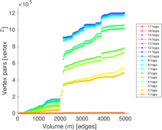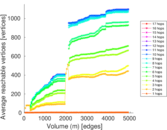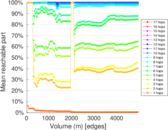### Diameter/density evolution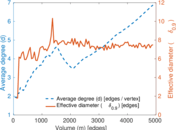### Matrix decompositions plots